# Texas Go Math Grade 1 Lesson 2.3 Answer Key Use Symbols to Compare

Refer to our Texas Go Math Grade 1 Answer Key Pdf to score good marks in the exams. Test yourself by practicing the problems from Texas Go Math Grade 1 Lesson 2.3 Answer Key Use Symbols to Compare.

## Texas Go Math Grade 1 Lesson 2.3 Answer Key Use Symbols to Compare

Essential Question
How can you use symbols to show how numbers compare?
Explanation:
We use inequality symbols to compare numbers. There are symbols for greater than, greater than or equal to, less than, and less than or equal to.

Explore
Use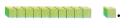Draw quick pictures to show your work. Write the numbers to compare.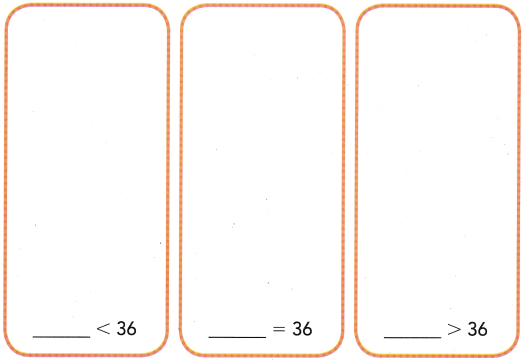Explanation:For The Teacher

• Have children use base-ten blocks to show a number less than 36, a number equal to 36, and a number greater than 36.
Explanation:
35 less than 36,
36 is a number equal to 36,
37  a number greater than 36.

Math Talk
Mathematical Processes
Compare 47 and 32 in two ways. What two symbols do you use? Explain
Explanation:
47 > 32 and
32 < 47
Greater than and less than 2 symbols are used

Model and Draw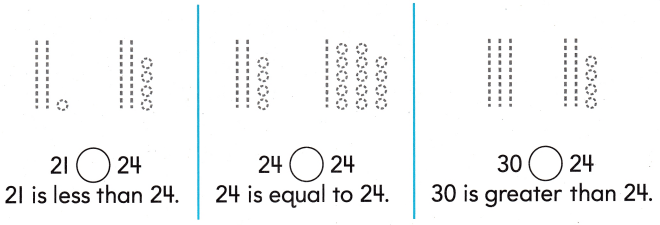Explanation: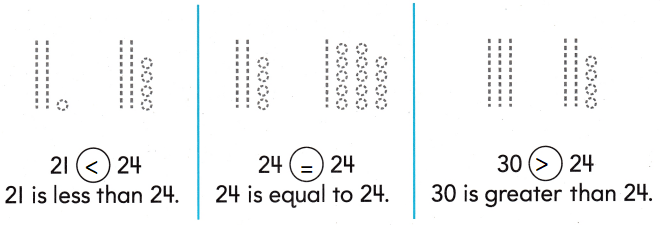Share and Show

Use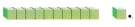Draw to show each number. Write <, >, or =. Complete the sentence.

Question 1.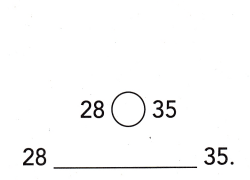Explanation:
Compared the tens if tens are equal
we have to compare the ones

Question 2.Explanation:
Compared the tens if tens are equal
we have to compare the ones

Question 3.Explanation:
Compared the tens if tens are equal
we have to compare the ones

Question 4.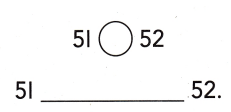Explanation:
Compared the tens if tens are equal
we have to compare the ones

Problem Solving
Write <, >, or =.

Draw a quick picture if you need to.

Remember
< is less than > is greater than = is equal

Question 5.
3743
37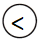43
Explanation:
Compared the tens if tens are equal
we have to compare the ones
37 is less than 43

Question 6.
6272
6272
Explanation:
Compared the tens if tens are equal
we have to compare the ones
62 is less than 72

Question 7.
6459
6459
Explanation:
Compared the tens if tens are equal
we have to compare the ones
64 is greater than 59

Question 8.
5656
56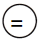56
Explanation:
Compared the tens if tens are equal
we have to compare the ones

Question 9.
6272
6272
Explanation:
Compared the tens if tens are equal
we have to compare the ones
62 is less than 72

Question 10.
7065
7065
Explanation:
Compared the tens if tens are equal
we have to compare the ones
70 is greater than 65

Question 11.
7984
7984
Explanation:
Compared the tens if tens are equal
we have to compare the ones
79 is less than 84

Question 12.
9093
9093
Explanation:
Compared the tens if tens are equal
we have to compare the ones
90 is less than 93

Question 13.
9390
9390
Explanation:
Compared the tens if tens are equal
we have to compare the ones
93 is greater than 90

Write or draw to solve.

Question 14.
Kate and Mia play a game. Kate has 27 cards. Mia has 31 cards. The fewer number of cards wins. Which number of cards wins this game?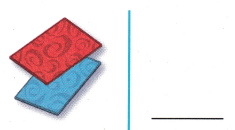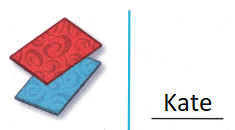Explanation:
Kate and Mia play a game. Kate has 27 cards. Mia has 31 cards.
The fewer number of cards wins.
As Kate has 27 cards. so Kate wins

Question 15.
H.O.T. Gill and Rob win tokens in a game. Gill has 86 tokens. Rob has 61 tokens. 70 tokens are needed for a prize. Who has enough tokens for a prize? Write the number.Explanation:
Gill and Rob win tokens in a game. Gill has 86 tokens. Rob has 61 tokens.
70 tokens are needed for a prize.
so, Gill wins the prize

Question 16.
H.O.T. Multi-Step Matt scores 34 points and wins the game. Lee scores points and does not win. The number of Lee’s points is greater than the number of Matt’s points. Is Lee’s score 49 or 29?
Explanation:
Matt scores 34 points and wins the game.
so, Lee score should be less
that is 29
29 < 34

Question 17.
The Titan ride in Texas holds 30 riders. Which number is less than 30?
(A) 33
(B) 29
(C) 45
Explanation:
Compared the tens if tens are equal
we have to compare the ones
29 < 30

Question 18.
A roller coaster can hold 32 riders. Which number of people can ride?
(A) 27
(B) 33
(C) 48Explanation:
Compared the tens if tens are equal
we have to compare the ones
27 < 32

Question 19.
Communicate A bus can hold 28 people. Which number is less than 28?
(A) 15
(B) 51
(C) 105
Explanation:
Compared the tens if tens are equal
we have to compare the ones
15 < 28

Question 20.
Texas Test Prep Which number is equal to 63?
(A) 36
(B) 63
(C) 81
Explanation:
Compared the tens if tens are equal
we have to compare the ones
63 = 63

Take Home Activity

• Have your child show you how to write <, >, and = to compare two numbers. Ask him or her to use words to explain each comparison.

### Texas Go Math Grade 1 Lesson 2.3 Homework and Practice Answer Key

UseDraw to show each number. Write <, >, or =. Complete the sentence.

Question 1.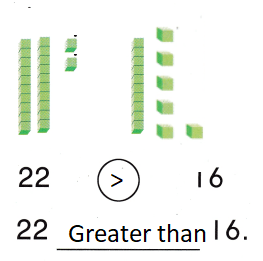Explanation:
Compared the tens if tens are equal
we have to compare the ones

Question 2.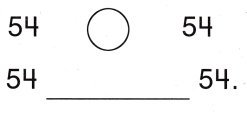Explanation:
Compared the tens if tens are equal
we have to compare the ones

Question 3.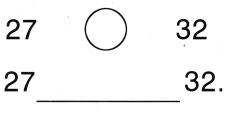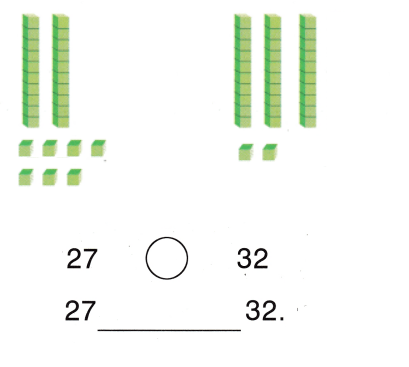Explanation:Compared the tens if tens are equal
we have to compare the ones

Question 4.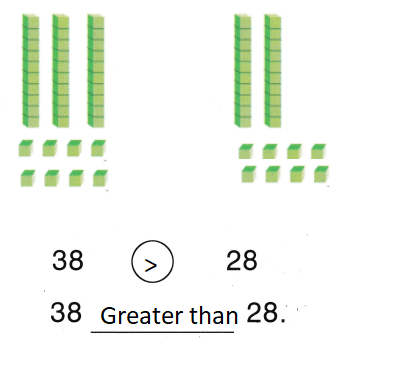Explanation:
Compared the tens if tens are equal
we have to compare the ones

Problem Solving

Write or draw to solve.

Question 5.
Noor and Gil spin a game wheel. Noor spins a 59. Gil spins a 79. It takes a 75 to win. Which number wins?
____
Gil wins the spin
Explanation:
Noor and Gil spin a game wheel.
Noor spins a 59. Gil spins a 79.
It takes a 75 to win.
so, Gil wins the spin

Texas Test Prep

Lesson Check

Question 6.
A school bus has 37 seats. Which number of people can ride in the bus?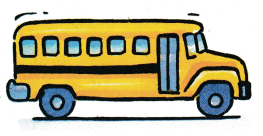(A) 41
(B) 37
(C) 39
Explanation:
Compared the tens if tens are equal
we have to compare the ones
37 = 37

Question 7.
Which number is greater than 82?
(A) 76
(B) 28
(C) 91
Explanation:
Compared the tens if tens are equal
we have to compare the ones
91 > 82

Question 8.
A box holds 4-5 dog treats. Which number is less than 45?(A) 51
(B) 13
(C) 54
Explanation:
Compared the tens if tens are equal
we have to compare the ones
13 < 45

Question 9.
Which number is greater than 22?
(A) 44
(B) 11
(C) 20
Explanation:
Compared the tens if tens are equal
we have to compare the ones
44 > 22

Question 10.
Which symbol is used to compare these numbers?
86 ___ 57
(A) =
(B) <
(C) >